• Call Now

1800-102-2727•

# Trigonometric Functions

There are six trigonometric functions: Sine function, Cosine function, Tangent function, Cosecant function, Secant function and Cotangent function. The details of each trigonometric functions is provided below:

## 1. Sinx function

f(x)= sin x is called sine function.

## Graph of Sinx function: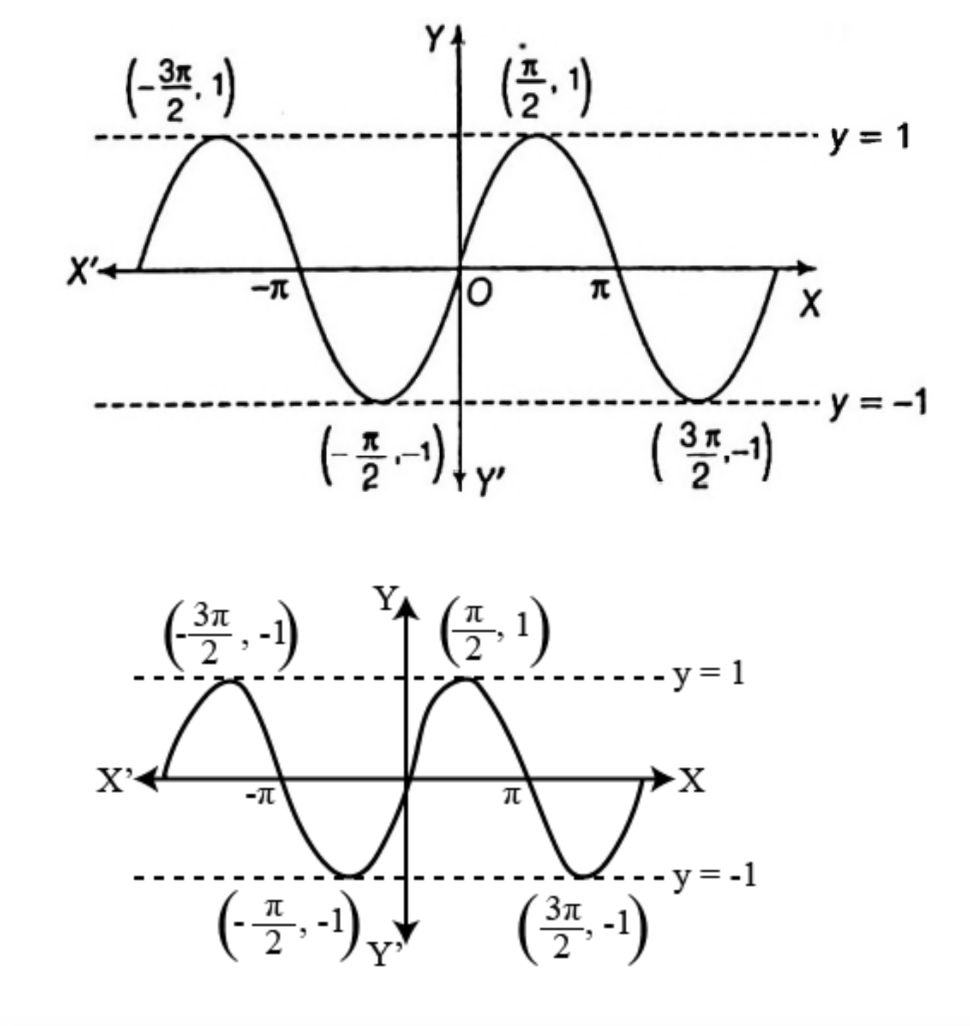## Domain and Range of Sinx function:

Domain of y=sin x is R and Range is [-1,1].

## Properties of Sine function:

• sin x is a periodic function having period 2,i.e., graph of sine function repeats after regular interval of length 2 units, so, y=sin x is a many-one function.
• Graph of y=sin x is symmetric about origin, so, sine function is called odd function.
• Sine function is bounded function as range of sine function lies between -1 to 1.

## 2. Cosine function

f(x)=cos x is called cosine function.

## Graph of Cosine function: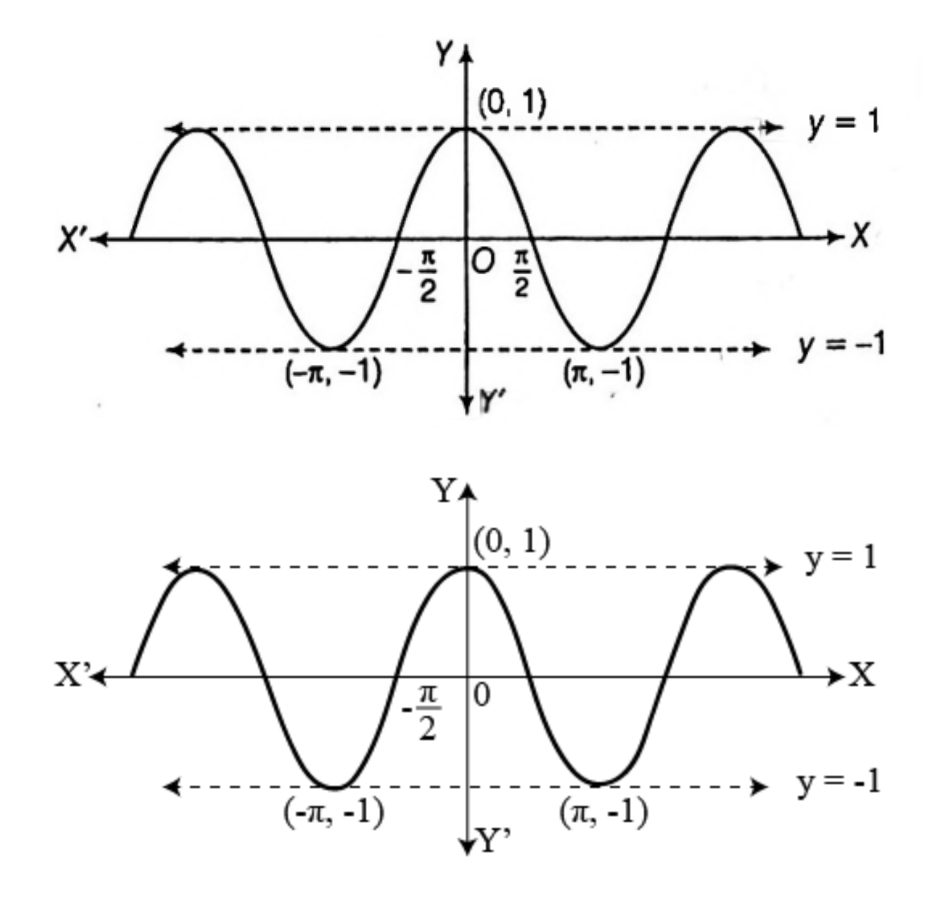## Domain and Range of Cosine function:

Domain of y=cos x is R and Range is [-1,1].

## Properties of Cosine function:

• cos x is a periodic function having period 2π,i.e., graph of cosine function repeats after regular interval of length 2π units, so, y=cos x is many-one function.
• Graph of y=cos x is symmetric about y-axis, so, cosine function is an even function.
• Cosine function is bounded function as range of cosine function lies between -1 to 1.

## 3.Tangent function

f(x)= tan x is called tangent function.

## Graph of Tangent function: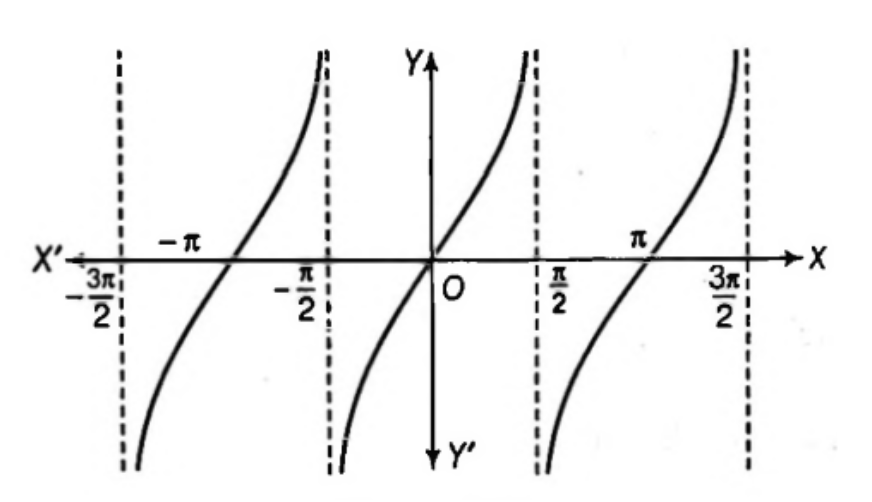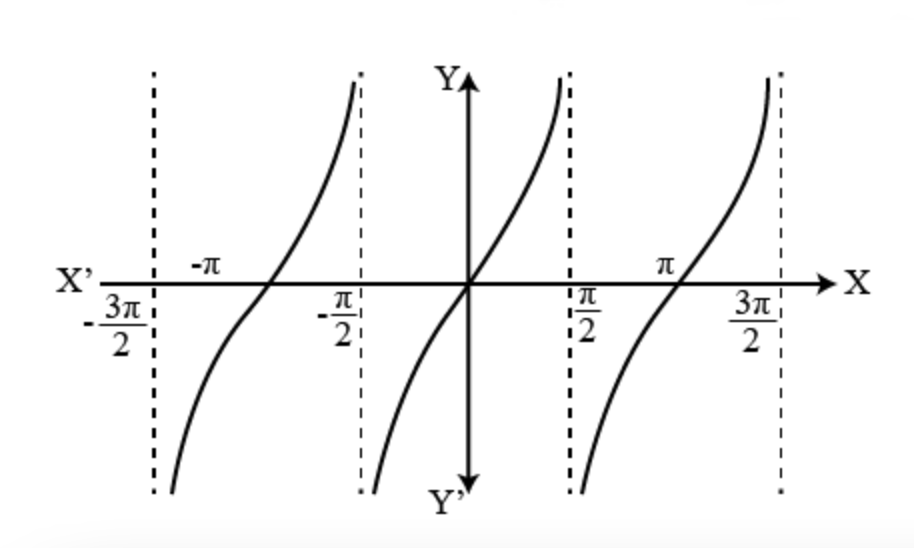## Domain and Range of tangent function:## Properties of tangent function:

• tan x is a periodic function having period π,i.e., graph of tangent function repeats after regular interval of length units, so, y=tan x is a many-one function.
• Graph of y=tan x is symmetric about origin, so, tangent function is an odd function.
• Tangent function is unbounded function as its range lies between -∞ to ∞ .

## 4. Cosecant function

f(x) = x is called cosecant function.

## Graph of Cosecant function: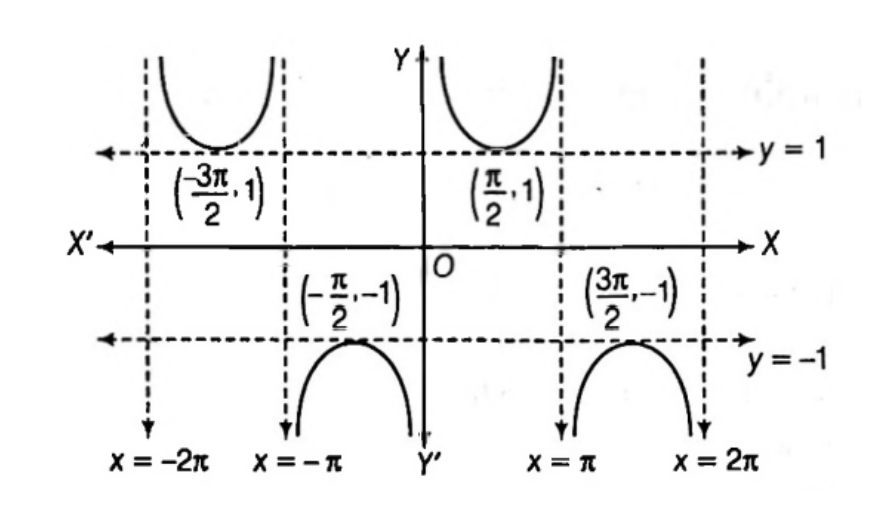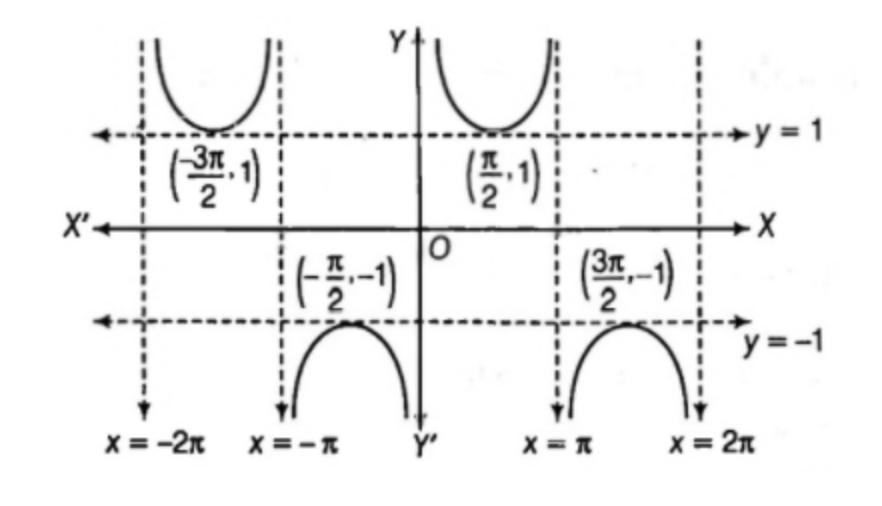## Domain and Range of Cosecant function:

Domain of y=x is R-nπ,n∈Z and Range is R-(-1,1).

## Properties of Cosecant function:

Domain of y=x is R-nπ,n∈Z and Range is R-(-1,1).

• Cosecant function & Sine function are reciprocal of each other ⇒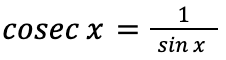• x is a periodic function having period 2π,i.e., graph of cosecant function repeats after regular interval of length 2π units, so, y=x is a many-one function.
• Graph of y=x is symmetric about origin, so, cosecant function is called odd function.
• Cosecant function is unbounded function as it can take any values except values lying between -1 to 1.

## 5. Secant function

f(x)=sec x is called secant function.

## Domain and Range of Secant function:## Properties of Secant function:

• Secant function & cosine function are reciprocal of each other ⇒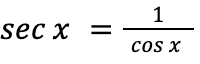• sec x is a periodic function having period 2π,i.e., graph of secant function repeats after a regular interval of length 2π units, so, y=sec x is a many-one function.
• Graph of y=sec x is symmetric about y-axis, so, secant function is an even function.
• Secant function is unbounded function as it can take any values except values lying between -1 to 1.

## 6. Cotangent function

f(x)=cot x is called cotangent function.

## Graph of Cotangent function: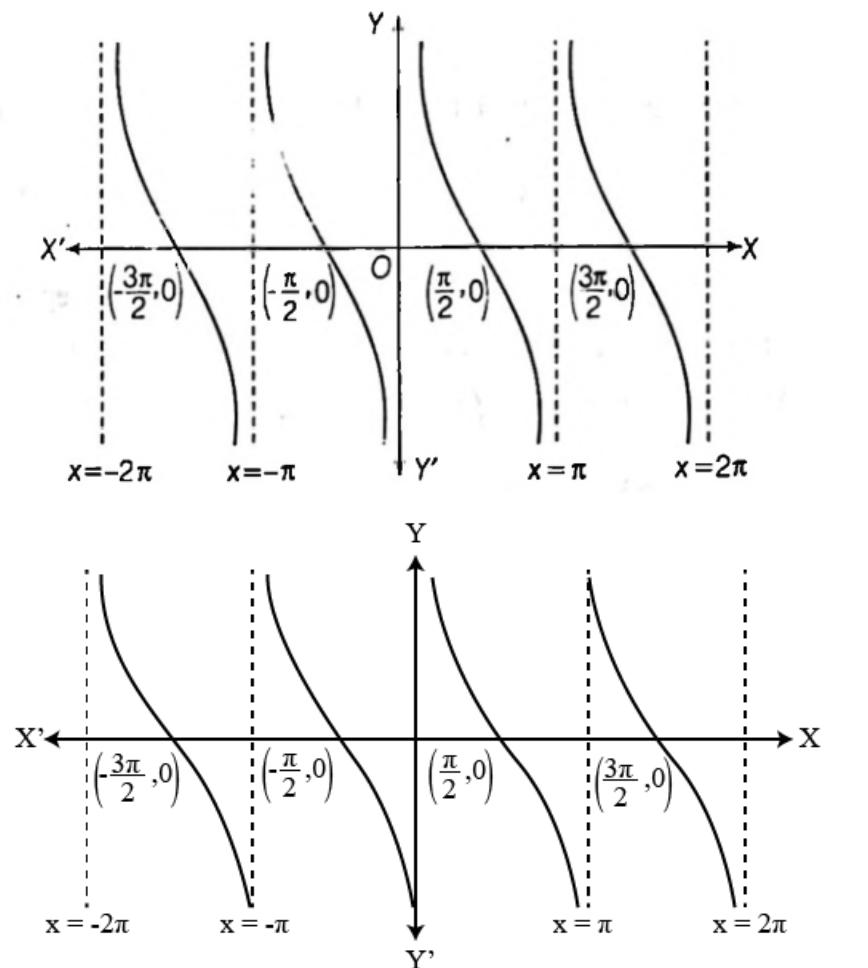## Domain & Range of Cotangent function:

Domain of y=cot x is R-nπ,n∈Z and Range is R.

## Properties of Cotangent function:

• Cotangent function & tangent function are reciprocal of each other ⇒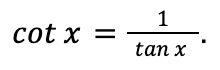• cot x is a periodic function having period π,i.e., graph of cotangent function repeats after a regular interval of length units, so, y=cot x is a many-one function.
• Graph of y=cot x is symmetric about origin, so, cotangent function is an odd function.
• Cotangent function is unbounded function as it can take any value lying between -∞ to ∞.

ALSO READ: Area of Triangle Formula

## Solved Examples:

Example 1: Find the domain of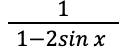Solution: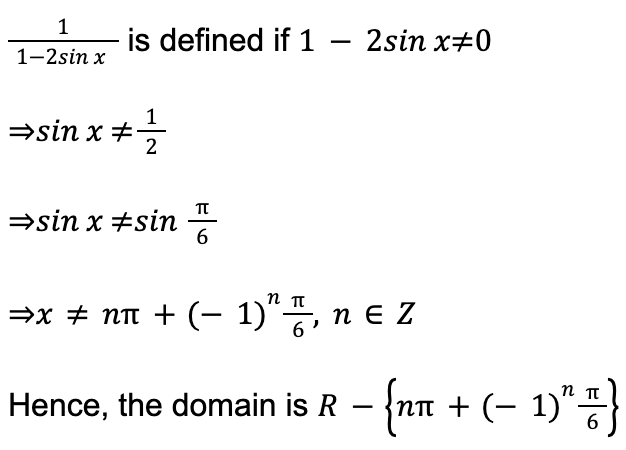Example 2: Find the range of f(x)=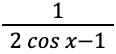Solution: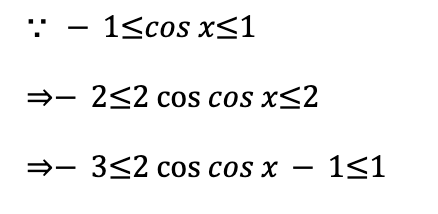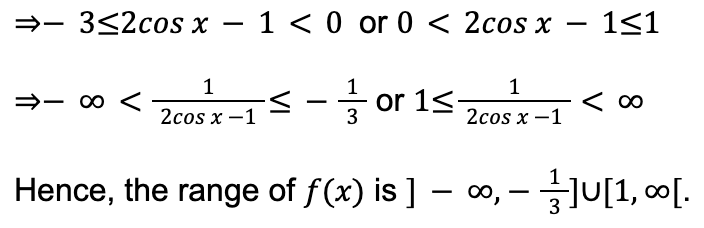Example 3: Find domain of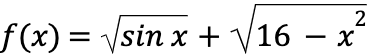Solution: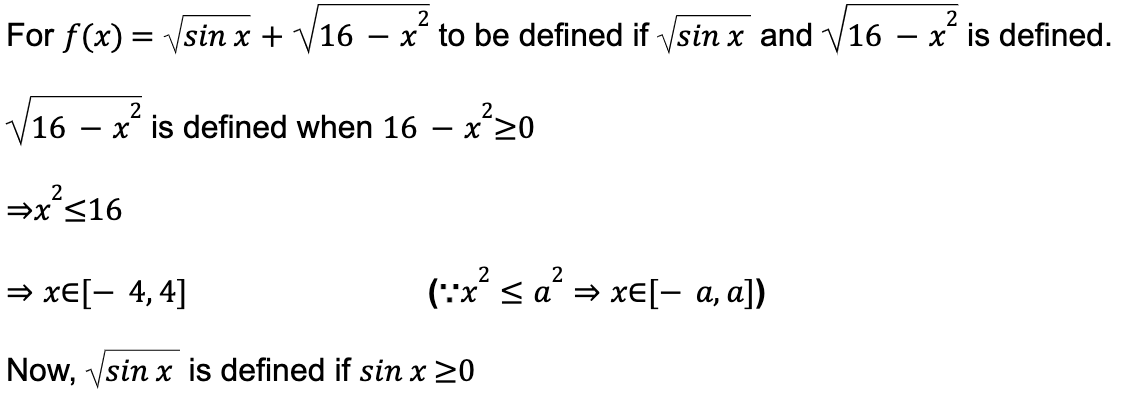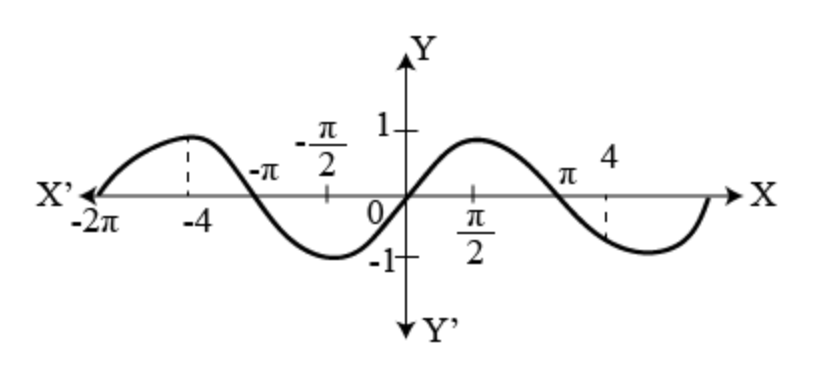Clearly, sin x ≥0, ∀x∈-4,-π∪[0,π] in the interval x∈[-4,4].

Hence, the domain of fx is x∈-4,-π∪[0,π]

## FAQs

1. What are the points where y=tan x is not defined.

Solution:

Since, the domain of y=tan x is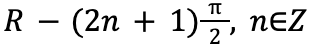So, y=tan x is not defined at odd multiples of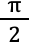.

2. What are the points where y=cot x is not defined.

Solution:

Since, the domain of y=cot x is R-nπ, n∈Z

So, y=cot x is not defined at integral multiples of π.

Pro Tip: Take a printout of the trigonometry table formula and pin it on your study wall for quick reference.

Talk to our expert
Resend OTP Timer =
By submitting up, I agree to receive all the Whatsapp communication on my registered number and Aakash terms and conditions and privacy policy